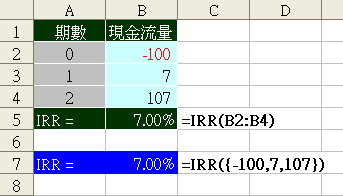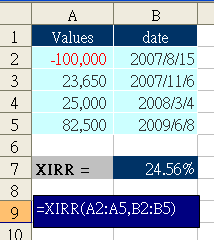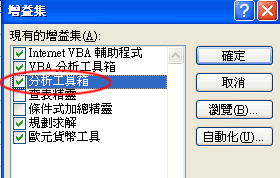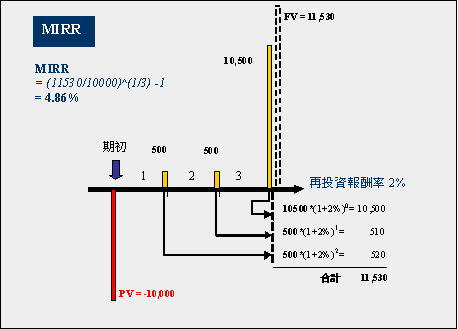# EXCEL財務函數IRR、XIRR、MIRR

﻿

IRR(Internal Rate of Return)稱為內部報酬率，其應用非常廣大，是學習財務管理不可或缺的工具。Excel也提供了一個相對函數IRR 來呼應。本篇也包含IRR相關的其他兩個函數XIRR以及MIRR，讓讀者可以清楚知道每個函數的應用時機。

# IRR函數0 = C0 + C1/(1+rate)1 + C2/(1+rate)2 + C3/(1+rate)3....+ Cn/(1+rate)n

IRR函數的參數定義如下：

=IRR(Values, guess)

 參數 意義 必要參數 Values 現金流量 必要 guess 猜測IRR可能的落點 選項

IRR的參數有兩個，一個是Values也就是『一序列』現金流量；另一個就是猜個IRR最可能的落點。那麼Value的值又該如何輸入？有兩種方式可輸入一序列的現金流量：

1. 使用陣列：例如=IRR({-100, 7, 107})，每一個數字代表一期的淨現金流量。
2. 儲存格的範圍：例如=IRR(B2:B4)，範圍中每一儲存格代表一期=IRR({-100, 7, 107})
=IRR(B2:B4)

## 使用者定義期間長短

IRR的參數並沒有絕對日期，只有『一期』的觀念。每一期可以是一年、一個月或一天，隨著使用者自行定義。如果每一格是代表一個『月』的現金流量，那麼傳回的報酬率就是『月報酬率』；如果每一格是代表一個『年』的現金流量，那麼傳回的報酬率就是『年報酬率』。

## guess-猜測報酬率可能的落點

guess真是個有趣的參數，IRR函數的任務不就是要解出報酬率的值嗎，怎會要我們自己猜測報酬率的落點呢？這不是很奇怪嗎，Excel計算功能那麼強，難道IRR函數無法直接解出來？沒錯IRR是無法解的。以{-100, -102, -104, -106, 450}這現金流量為例，等於得求出下列方程式中rate的解：

0 = -100 -102/(1+rate)1 -104/(1+rate)2 -106/(1+rate)3 + 450/(1+rate)4

=IRR({-100, -102, -104, -106, 450})
=IRR({-100, -102, -104, -106, 450}, 1%)
=IRR({-100, -102, -104, -106, 450}, 2%)

### guess是選項參數

guess參數可以省略不輸入，這時Excel會使用預設值10%。通常這是一年為一期報酬率都落在這附近，如果要計算月報酬率最好輸入1%，依此類推。

# XIRR函數

 日期 金額 2007/8/15 -100,000 2007/11/6 23,650 2008/3/4 25,000 2009/6/8 82,500

## XIRR參數

XIRR(values, dates, guess)

 參數 意義 必要參數 Values 現金流量的值 必要 dates 現金流量發生日期 必要 guess 猜測XIRR可能的落點 選項## 需要開啟分析工具箱

1) 工具/增益集
2) 將分析工具箱打勾
3) 按確定# MIRR函數

## MIRR參數

MIRR(values, finance_rate, reinvest_rate)

 參數 意義 必要參數 values 現金流量的值 必要 finance_rate 融資利率 必要 reinvest_rate 再投資報酬率 必要

MIRR是Modified Internal Rate of Return的縮寫，意思是改良式的IRR。IRR到底有何缺點，需要去修正呢？主要的原因是IRR並未考慮期間領回現金再投資問題！IRR的現金流量裡可分為正值及負值兩大類，正值部分屬於投資期中投資者拿回去的現金，這些期中拿回去的現金該如何運用，會影響報酬率的。負值部分屬於投資期中額外再投入的資金，這些資金的取得也有融資利率方面要考慮。

MIRR使用的方式是將期間所有的現金流入，全部以『再投資利率』計算終值FV。期間所有的現金流出，全部以『融資利率』計算現值PV。那麼MIRR的報酬率：

=(FV/PV)1/n - 1## 再投資報酬率

=IRR({-10000, 500, 500, 10500}) = 5%

= 500*(1+2%) + 500*(1+2%)2 +10500 ( 以Excel 表示 =500*(1+2%)+500*(1+2%)^2 + 10500 )
= 11,530

= (11530/10000)1/3-1 (Excel 表示 =(11530/10000)^(1/3)-1 )
= 4.86%

MIRR可以不用那麼麻煩，只需輸入再投資報酬率2%，便可輕易得到實際報酬率：
= MIRR(({-10000, 500, 500, 10500}), 0, 2%)
= 4.86%= MIRR(({-10000, 500, 500, 10500}), 0, 4%)
= 4.95%

= MIRR(({-10000, 500, 500, 10500}), 0, 5%)
= 5.00%

## 融資利率

= MIRR({-50000, -50000, 30000, 30000, 30000, 30000}, 5%, 3%)
= 5.15%=50000 + 50000/(1+5%)
=97,619

= 30000 + 30000*(1+3%) + 30000*(1+3%)^2 + 30000*(1+3%)^3
=125,508

= (125508/97619)^(1/5)-1
= 5.15%

『融資利率』是微軟的說明所使用的名詞，我認為較為適當的應該是『資金報酬率』(finance_rate)，也就是資金存放標的之報酬率。

# 相關文章

EXCEL投資理財應用

﻿

■ 本網站內容儘可能精確完整，但不保證無誤。若做為投資依據，風險請自行斟酌 ，本網站不負賠償之責任。
■ 網站所有資料均為版權所有，非經書面允許請勿轉載或使用。